General formulaeNext: Commentary Up: No Title Previous: Morlet transform of

# General formulae

It may be useful at this point to summarize the key formulae of wavelet transforms. Let us call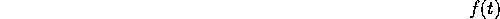the signal,the wavelet,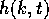the wavelet transform. Complex conjugation is denoted by an asterisk. The Fourier transform of a function is denoted by capital letters, and is defined in general as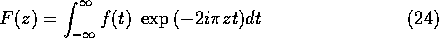Wavelet is called admissible if its norm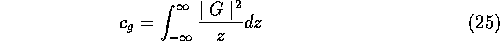is finite. For a real-valued continuous wavelet, it can be shown that this implies the relationUnder this condition, we have the definition of the wavelet transform: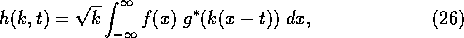the inverse transform formulaand the Parseval formula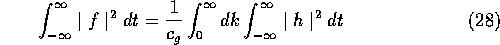Jacques Lewalle
Mon Nov 13 10:51:25 EST 1995